# High School Chemistry : Compounds and Bonding

## Example Questions

### Example Question #21 : Compounds And Bonding

What is the correct name for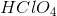?

Hydrochloric acid

Chloric acid

Perchloric acid

Chlorous acid

None of the answer choices are correct

Perchloric acid

Explanation:consists of a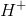ion bonded to a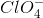ion, which is a perchlorate ion. Hence, the name of the ion's corresponding acid would be perchloric acid.

### Example Question #22 : Compounds And Bonding

What is the name of the following compound: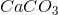?

calcium carbonate

calcium monocarbon trioxide

calcium carbonite

calcium trioxide

calcium carbon

calcium carbonate

Explanation:

In this case, we have an ionic compound. Calcium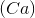is bound ionically to the polyatomic ion, carbonate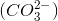. Thus, the name of this compound is calcium carbonate.

### Example Question #23 : Naming Compounds

What is the name for the following compound: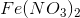?

Iron(II) nitrite

Iron(I) nitrate

Iron(II) nitrate

Iron(I) nitrite

Iron nitrate

Iron(II) nitrate

Explanation:

This is an ionic compound because there is a metal (iron) bound to a nonmetal (the polyatomic ion nitrate). Iron,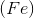is a transition metal, which means it can take on multiple different charges. To find the charge of iron in this compound we must not that the compound as a whole is neutral. Also we must know the charge on the nitrate ion,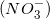, is negative one. To balance the two nitrate ions with one iron atom, the iron must carry a positive two charge. In naming ionic compounds with transition metals, we must use Roman numerals to indicate the charge on the metal. Thus the name of this compound is iron(II) nitrate.

### Example Question #23 : Compounds And Bonding

Choose the correct chemical formula for lead (II) chloride.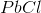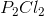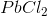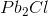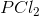Explanation:is the correct answer because it takes two chloride ions to balance the positive two charge on the lead atom. lead (II) refers to the ion of lead with a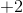charge instead of Lead (IV) which refers to the ion with a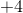charge. Note that roman numerals are used with elements that can form more than one cation (generally transition metals).

### Example Question #1 : Help With Polyatomic Ions

Which of the following polyatomic ions has a net charge of?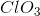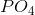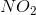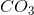Explanation:

For this question, you need to have the charges of polyatomic ions memorized. This will tell you that of the answer choices only the carbonate ion has a net charge of.

Chlorate: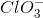Nitrite: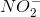Phosphate: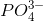Carbonate: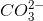### Example Question #2 : Help With Polyatomic Ions

When the ionic compound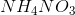is put in water, it dissociates to produce which of the following ions?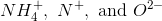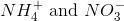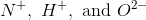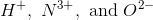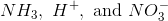Explanation: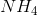and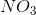are known as polyatomic ions. When a compound made up of polyatomic ions ionizes in water, it separates into the original polyatomic ions, not the individual elements.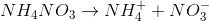### Example Question #3 : Help With Polyatomic Ions

What is the correct molecular formula for phosphoric acid?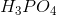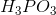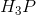None of the answer choices are correctExplanation:

Phosphoric acid combines a single phosphate ion with three hydrogen ions. The correct molecular formula is.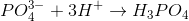### Example Question #1 : Help With Molecular Formulas

Choose the correct chemical formula for sodium sulfate.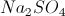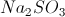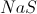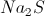Explanation:

Sulfate is a polyatomic ion that is composed of one sulfur atom and four oxygen atoms. The ion has a net charge of.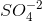Since sodium ions have a charge of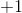, we must use two atoms of sodium in order to result in a neutrally charged compound.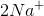This makes the neutral chemical compound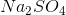.

### Example Question #2 : Help With Molecular Formulas

An unknown compound has the following composition:

40% carbon

6.7% hydrogen

53.3% oxygen

If the molecular formula for this compound is six times as heavy as the empirical formula, what is the molecular formula for the compound?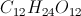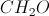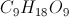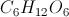Explanation:

Since we know the percentages by mass, we can convert them into grams by imagining a 100-gram sample of the mystery compound. At that point, we can determine how many moles are present for each element: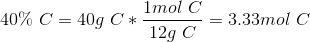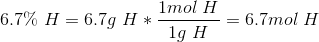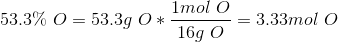By dividing each molar amount by the smallest molar amount (in this case 3.33), we can find the elemental ratios.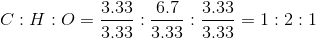This means that the empirical formula for this compound is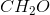.

Since we were told that the molecular formula is six times as heavy as the empirical formula, we need to multiply each elemental amount in the empirical formula by six. After doing this, we find that the molecular formula is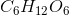.

### Example Question #3 : Help With Molecular Formulas

What specific functional group is created when a carbon atom forms a double bond with an oxygen atom and a single bond with an -OH group?

Carboxyl group

alcohol

Carbonyl group

Ester

Ether

Carboxyl group

Explanation:

Carboxylic acids are formed by a carbon atom with a double bond to an oxygen atom, as well as an -oh substituent. This leaves one remaining bond for the carbon atom, allowing it to bind to a larger molecular component. The name for a carboxylic acid bound to a larger molecule is a "carboxyl group."

Carbonyl groups are formed when a carbon atom forms a doubl bond with an oxygen atom and any two other substituents. Carboxylic acids, aldehydes, ketones, esters, and amides are all carbonyl functional groups.

An ester is formed when a carbon forms a double bond with an oxygen and a single bond with a second oxygen atom. The second oxygen is generally incorporated into the backbone structure of the molecule, rather than as a single substituent to the carbon.

An ether is formed by an oxygen atom bound to two other atoms (usually carbons).

### All High School Chemistry Resources